## Addition of five digit numbers without regrouping

Question 1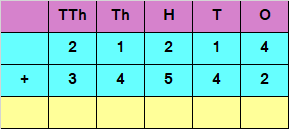Explanation

Adding the digits at ones place,

4 + 2 = 6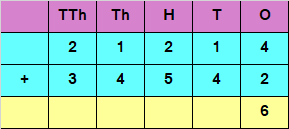Adding the digits at tens place

1 + 4 = 5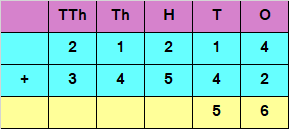Adding the digits at hundreds place

2 + 5 = 7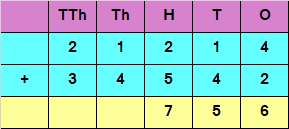Adding the digits at thousands place

1 + 4 = 5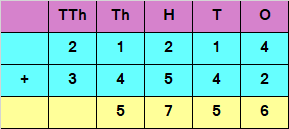Adding the digits at ten thousands place

2 + 3 = 5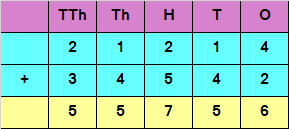Hence, the sum of the given numbers is 55756

Question 2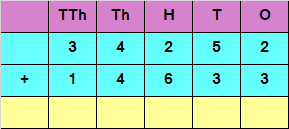Explanation

Adding the digits at ones place

2 + 3 = 5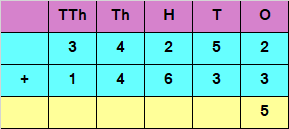Adding the digits at tens place

5 + 3 = 8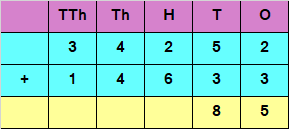Adding the digits at hundreds place

2 + 6 = 8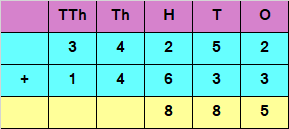Adding the digits at thousands place

4 + 4 = 8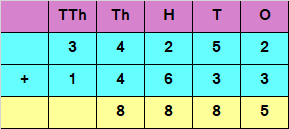Adding the digits at ten thousands place

3 + 1 = 4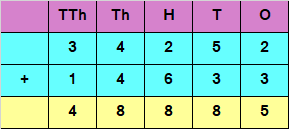Hence, the sum of the given numbers is 48885

## Addition of five digit number with regrouping

Question 1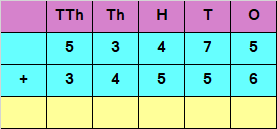Explanation

Adding the digits at ones place

5 + 6 = 11

Since the number is more than 9, we carry over 1 to the tens place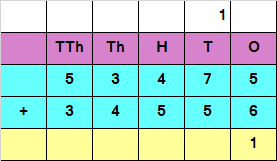Adding the digits at tens place and the carry of 1

7 + 5 + 1 = 13

Since the number is more than 9, we carry over 1 to the hundreds place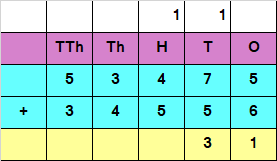Adding the digit at hundreds place and the carry of 1

4 + 5 + 1 = 10

Since the number is more than 9, we carry over 1 to the thousands place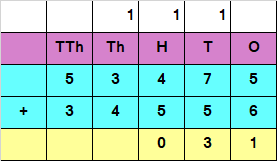Adding the digits at thousands place and the carry of 1

3 + 4 + 1 = 8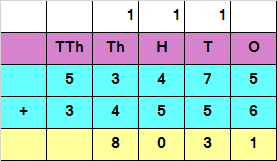Adding the digits at ten thousands place

5 + 3 = 8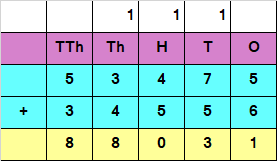Hence, the sum of the given numbers is 88031.

Question 2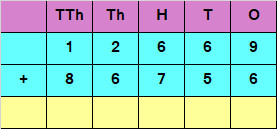Explanation

Adding the digits at ones place

9 + 6 = 15

Since the number is more than 9, we carry over 1 to the tens place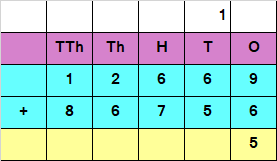Adding the digits at tens place and the carry of 1

6 + 5 + 1 = 12

Since the number is more than 9, we carry over 1 to the hundreds place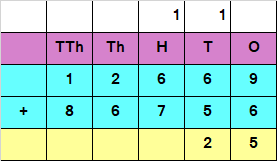Adding the digits at hundreds place and the carry of 1

6 + 7 + 1 = 14

Since the number is more than 9, we carry over 1 to the thousands place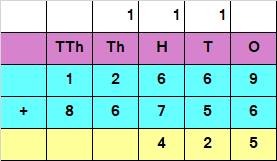Adding the digits at thousands place and the carry of 1

2 + 6 + 1 = 9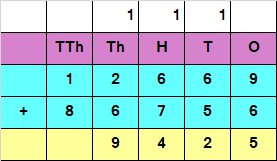Adding the digits at ten thousands place

1 + 8 = 9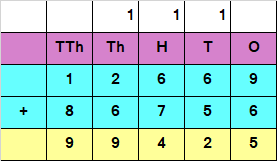Hence, the sum of the given numbers is 99425